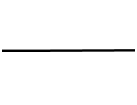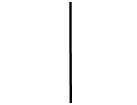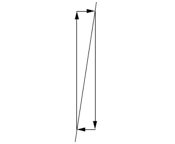﻿ Practice With Slope
PRACTICE WITH SLOPE

In a rush? Need to skip the concepts and jump right to the slope formula?
(Please come back and explore the beautiful ideas at a later time.)

Consider the equation $\,y = mx + b\,$.
As we'll see in this section, every equation of this form graphs as a non-vertical line in the coordinate plane.

In this equation, $\,m\,$ is the coefficient of the $\,x$-term, and $\,b\,$ is a constant term.
For example, in the equation $\,y = 2x + 3\,$, we have $\,m = 2\,$ and $\,b = 3\,$.

The number $\,m\,$ is called the slope of the line, and gives information about the ‘slant’ of the line.
It answers questions like:   “Is it an uphill or downhill line?   How steep is it?”
The purpose of this section is to explain why this is true.

Why does $\,y = mx + b\,$ graph as a line?
Why does the number $\,m\,$ give info about the slant of this line?
 The key idea (which we'll prove below) is that the equation $\,y = mx + b\,$ defines a very special relationship between $\,x\,$ and $\,y\,$: equal changes in $\,x\,$ (the input) give rise to equal changes in $\,y\,$ (the output). Indeed, if $\,x\,$ changes by an amount $\,\Delta x\,$ (read aloud as ‘delta ex’ or ‘change in ex’), then $\,y\,$ changes by $\,m\Delta x\,$. That is, $\,y\,$ changes $\,m\,$ times as fast as $\,x\,$. This is a bit symbol-intensive, so let's look at a concrete example. Suppose that $\,m\,$ is $\,2\,$. (See the sketch at right.) Then, $\,y\,$ changes $\,2\,$ times as fast as $\,x\,$ (that is, twice as fast as $\,x\,$). If (say) $\,x\,$ changes by $\,1\,$, then $\,y\,$ will change by $\,2(1) = 2\,$. If (say) $\,x\,$ changes by $\,5\,$, then $\,y\,$ will change by $\,2(5) = 10\,$, and so on. Here's another way to look at it. Imagine you're ‘standing on’ a point in the coordinate plane. Let's force $\,y\,$ to change twice as fast as $\,x\,$, and see what new points result: If you take $\,1\,$ step to the right (i.e., let $\,x\,$ change by $\,1\,$), then you'll have to take $\,2\,$ steps up (i.e., let $\,y\,$ change by $\,2\,$). Do it again—one step to the right, two steps up. Do it again—one step to the right, two steps up. (Repeat, if you want, with different changes in $\,x\,$.) Hmmm$\,\ldots\,$ what pattern are these points creating? A line!! If we repeat the previous exercise with $\,m=100\,$, think about the line that would result: one step to the right, $\,100\,$ steps up. Pretty steep line! So, clearly, the number $\,m\,$ gives information about the steepness or ‘slant’ of the line.The next part is a bit technical, so perhaps a break is in order first!

PROOF THAT, IN THE EQUATION $\,y=mx+b\,$,
EQUAL CHANGES IN $\,x\,$ GIVE RISE TO EQUAL CHANGES IN $\,y\,$

Let $\,(x_1,y_1)\,$ be a point on the graph of $\,y = mx + b\,$, so that $\,y_1 = mx_1 + b\,$.
That is, when you substitute $\,x_1\,$ for $\,x\,$ and $\,y_1\,$ for $\,y\,$, the resulting equation is true.

Let $\,x_1\,$ change by an amount $\,\Delta x\,$, to get a new $\,x\,$-value:   $\,x_2 = x_1 + \Delta x\,$

Let $\,y_2\,$ represent the $\,y$-value corresponding to $\,x_2\,$.
Then,
 $y_2 = mx_2 + b$ (find the $\,y$-value corresponding to $\,x_2\,$;   call it $\,y_2\,$) $= m(x_1 + \Delta x) + b$ (substitute $\,x_1 + \Delta x\,$ for $\,x_2\,$) $= mx_1 + m\Delta x + b$ (multiply out; i.e., use the Distributive Law) $= (mx_1 + b) + m\Delta x$ (re-order and re-group) $= y_1 + m\Delta x$ (look back:   $\,mx_1 + b\,$ is precisely $\,y_1\,$)
Thus, $\,y\,$ has changed by $\,m\Delta x\,$.

Make sure you see this!
 The starting point is: $($ $x_1$ $,$ $y_1$ $)$ The ending point is: $($ $x_1 + \Delta x$ $,$ $y_1 + m\Delta x$ $)$
So, when $\,x\,$ changes by $\,\Delta x\,$, $\,y\,$ changes by $\,m\Delta x\,$.

A FORMULA FOR SLOPE

Let's summarize things from the previous proof.
We started at $\,(x_1,y_1)\,$.
We ended at $\,(x_2,y_2)\,$.

The change in $\,x\,$ is:   $\,\Delta x = x_2 - x_1\,$
A ‘change in $\,x\,$’ is informally called a run, since it is in the left/right direction.
Memory device:   Run to the right! Run to the left!

The change in $\,y\,$ is:   $\,\Delta y = y_2 - y_1\,$
A ‘change in $\,y\,$’ is informally called a rise, since it is in the up/down direction.
Memory device:   Rise up!

And, we learned that the change in $\,y\,$ is $\,m\Delta x\,$:   in equation form, $\,\Delta y = m\Delta x\,$.
Solving this last equation for the slope, $\,m\,$, gives us an important formula:

SLOPE OF A LINE
Let $\,(x_1,y_1)\,$ and $\,(x_2,y_2)\,$ be two different points on a non-vertical line.
Then, the slope of this line is: $$m = \text{slope} = \frac{y_2-y_1}{x_2-x_1} = \frac{\Delta y}{\Delta x} = \frac{\text{change in } y}{\text{change in } x} = \frac{\text{rise}}{\text{run}}$$
ANALYSIS OF THE SLOPE FORMULA

There are lots of important things you should know about the slope formula:

• HOW TO USE THE SLOPE FORMULA:
To find the slope of a non-vertical line, you can choose any two different points on the line.
Take the difference of the $\,y$-values. This is your ‘rise’.
Take the difference of the $\,x$-values. This is your ‘run’.
Divide rise by run, and you've got your slope!
• BE CAREFUL—ORDER IS IMPORTANT!
Once you do your first subtraction, the next subtraction must be done in the same order.
For example, suppose your two points are $\,(1,5)\,$ and $\,(3,12)\,$.
If you say $\,\Delta x = 3-1=2\,$, then you must say $\,\Delta y = 12-5 = 7\,$, and $\,m = \frac{7}{2}\,$.
Or:
If you say $\,\Delta x = 1-3=-2\,$, then you must say $\,\Delta y = 5-12 = -7\,$, and $\,m = \frac{-7}{-2} = \frac 72\,$.
Either way is fine.
But DON'T use (say) $\,3-1\,$ for one and $\,5-12\,$ for the other!
• RUN:
If the change in $\,x\,$ is $\,2\,$, you go to the right two.
If the change in $\,x\,$ is $\,-2\,$, you go to the left two.
‘Run’ is positive to the right, and negative to the left.
• RISE:
If the change in $\,y\,$ is $\,2\,$, you go up two.
If the change in $\,y\,$ is $\,-2\,$, you go down two.
‘Rise’ is positive UP, and negative DOWN.
• HORIZONTAL LINES:
Since two points on a horizontal line have the same $\,y$-value,
$\,\text{slope} = \frac{y_2-y_1}{x_2-x_1} = \frac{0}{x_2-x_1} = 0\,$.
The slope of a horizontal line is zero.
• VERTICAL LINES:
Since two points on a vertical line have the same $\,x$-value,
an attempt to compute the slope fails:
$\,\text{slope} = \frac{y_2-y_1}{x_2-x_1} = \frac{y_2-y_1}{0} = \text{Can't continue!}$
Division by zero is not allowed.
Thus, you can't compute the slope of a vertical line.
We say that vertical lines have NO slope.
• ZERO SLOPE versus NO SLOPE:
Note that ‘zero slope’ and ‘no slope’ mean two entirely different things!
Horizontal lines have zero slope; vertical lines have no slope.horizontal line,zero slope vertical line,no slope
• UPHILL LINES, POSITIVE SLOPE:
Imagine you're standing on a line in the coordinate plane, walking from left to right.
If you're going uphill, then we'll informally call this an ‘uphill line’.
On uphill lines, if you rise-and-run from point-to-point, one of two situations occur:
• rise and run are both positive, so $\text{slope} = \ \frac{+}{+}\ = \text{positive}$
• rise and run are both negative, so $\text{slope} = \ \frac{-}{-}\ = \text{positive}$
Thus, uphill lines have positive slopes.
 UPHILL LINES, POSITIVE SLOPESgradual uphill,small positive slope steep uphill,large positive slope
 DOWNHILL LINES, NEGATIVE SLOPESgradual downhill,small negative slope steep downhill,large negative slope
• DOWNHILL LINES, NEGATIVE SLOPE:
Imagine you're standing on a line in the coordinate plane, walking from left to right.
If you're going downhill, then we'll informally call this a ‘downhill line’.
On downhill lines, if you rise-and-run from point-to-point, one of two situations occur:
• rise is positive and run is negative, so $\text{slope} = \ \frac{+}{-}\ = \text{negative}$
• rise is negative and run is positive, so $\text{slope} = \ \frac{-}{+}\ = \text{negative}$
Thus, downhill lines have negative slopes.
EXAMPLES:
Question:
Find the slope of the line through $\,(-1,3)\,$ and $\,(2,-5)\,$.
Solution:
$\displaystyle\text{slope} = \frac{-5-3}{2-(-1)} = \frac{-8}{3} = -\frac83$

The answers in this exercise are reported as fractions, in simplest form.
Question:
Find the slope of the line through $\,(2,5)\,$ and $\,(-7,5)\,$.
Solution:
$\displaystyle\text{slope} = \frac{5-5}{-7-2} = \frac{0}{-9} = 0$

Recall that zero, divided by any nonzero number, is zero.
This is a horizontal line.
Question:
Find the slope of the line through $\,(2,5)\,$ and $\,(2,-7)\,$.
Solution:
$\displaystyle\text{slope} = \frac{-7-5}{2-2} = \frac{-12}{0} = \text{can't continue}$

Division by zero is not allowed.
This line has no slope.
It is a vertical line.
Question:
Horizontal lines have (circle one):    no slope        zero slope
Solution:
zero slope
Question:
Vertical lines have (circle one):    no slope        zero slope
Solution:
no slope
Question:
Suppose a fraction has a zero in the numerator, and a nonzero denominator.
What is the value of the fraction?
Solution:
zero
Question:
Suppose a fraction has a zero in the denominator, and a nonzero numerator.
What is the value of the fraction?
Solution:
it is not defined
Question:
Start at a point $\,(x,y)\,$ on a line.
To get to a new point, move up $\,3\,$ and to the right $\,4\,$.
What is the slope of the line?
Solution:
Rise is $\,3\,$;   run is $\,4\,$.
$\displaystyle\text{slope} = \frac{\text{rise}}{\text{run}} = \frac{3}{4}$
Question:
Start at a point $\,(x,y)\,$ on a line.
To get to a new point, move down $\,2\,$ and to the left $\,6\,$.
What is the slope of the line?
Solution:
Rise is $\,-2\,$;   run is $\,-6\,$.
$\displaystyle\text{slope} = \frac{\text{rise}}{\text{run}} = \frac{-2}{-6} = \frac13$
Question:
Start at a point $\,(x,y)\,$ on a line.
To get to a new point, move straight up $\,5\,$ units.
What is the slope of the line?
Solution:
no slope (vertical line)
Question:
Start at a point $\,(x,y)\,$ on a line.
To get to a new point, move directly to the left $\,5\,$ units.
What is the slope of the line?
Solution:
zero slope (horizontal line)
Question:
Suppose you are walking along a line, moving from left to right.
You are going uphill.
Then, the slope of the line is (circle one):    positive        negative
Solution:
positive
Master the ideas from this section

When you're done practicing, move on to:
Graphing Lines

CONCEPT QUESTIONS EXERCISE: